# You need an equation

### Insert chemical equations in Notes

Chemical equation fields (Chembox) allow easy entry of chemical formulas and equations such as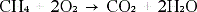.

When entering into a Chembox, most of the formatting work is automatically done for you:

 • The symbols of most elements, such as Ag and Cl, are automatically case-sensitive.
 • Leading digits are treated as coefficients and are displayed in full size. Numbers that follow an element or a closed bracket appear in subscript.
 • The equal sign ("=") is converted into a reaction arrow (&).

Hints:

 • Equations in a Chembox cannot be calculated or balanced.
 • The automatic upper and lower case of the elements does not work in individual cases. For example, when entering carbon dioxide (CO2), you have to capitalize the "O" by hand. Otherwise, entering “co” would result in the symbol “Co” and thus cobalt.

### Entering a chemical equation

 1 Place the cursor where you want to place the equation in the Notes workspace.
 2 From the Insert menu, choose Chembox. - or - Press the key combination Ctrl + E (Mac®: Press “+ E).

An empty field for chemical equations appears.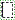3 Enter the equation in the box. For sulfuric acid you need e.g. B. Enter h2sO4 and capitalize the O itself.

The Chembox automatically formats the text as you type: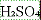4 If you need superscript characters for ion equations, first enter a circumflex (^) and then the text.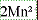5 Use parentheses to indicate whether a compound is solid (s), liquid (l), gas (g), or aqueous (aq).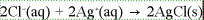6 To exit the Chembox, click an area outside.